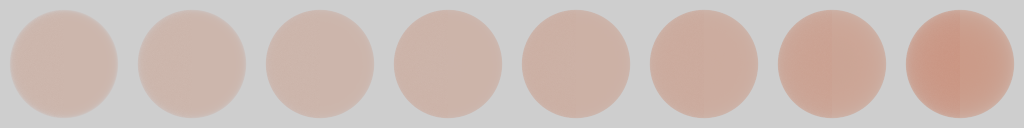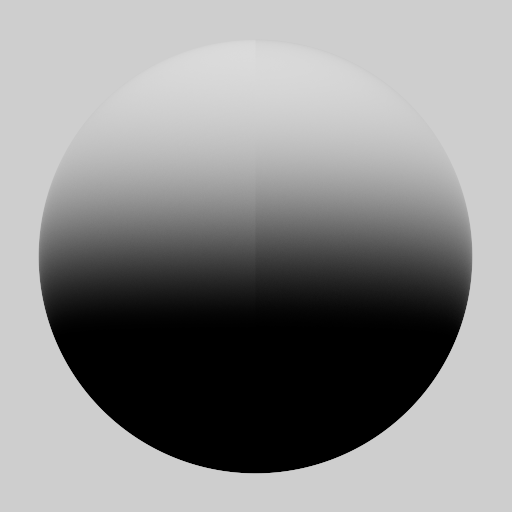# A Multi-Faceted Exploration (Part 3)## A New Dimension

In the last post, I’d shown how a small tweak to Imageworks’ multiple-scattering Fresnel term, $\color{brown}{F_\mathrm{ms}}$, brought their approximation closer to the reference solution of Heitz et al. However, there was still a niggling difference at high roughness, most noticeable under uniform lighting:Figure 1: Furnace test, with roughness $\in [\frac{1}{8}, \frac{2}{8}, \ldots 1]$ (left halves: Imageworks, right halves: Heitz).

At the end of the day, $\color{brown}{F_\mathrm{ms}}$ is based on a simple diffuse model, and its limitations become more apparent as multiple scattering increases with roughness.

A more accurate alternative would be to precalculate a multiple-scattering directional albedo LUT that incorporates Fresnel, directly from the Heitz model. This new term, which I’ll call $\color{teal}{E_\mathrm{Fms}}$, leads to the following minor change to the multiple-scattering lobe:

The good news is that this version produces results that match Heitz in a furnace environment:Figure 2: Furnace test, with roughness $\in [\frac{1}{8}, \frac{2}{8}, \ldots 1]$ (left halves: with $\Ems$, right halves: Heitz).

The bad news is that we need a 3D LUT for $\Ems$, since it depends not only on the view angle ($\mu_o$) and roughness, but also $\Fr$ (or specular reflectance). Worse, for coloured metals – such as our lovely copper example – we’d need to do three separate 3D lookups for R, G and B. The only silver lining is that this cost can potentially be amortised across lights, but it’s still less than ideal for real-time applications.

Note: a secondary concern is that this change also breaks the recipriocity of the multiple-scattering model, since the results will be different if $\mu_o$ and $\mu_i$ are swapped. While this isn’t a practical issue for real-time rendering, it could be a problem in other contexts (e.g. bidirectional path tracing).

## Schlick Alternative

If we’re willing to restrict ourselves to Schlick’s Fresnel approximation1, then we can adapt a popular approach that’s been used with environmental lighting in games for a number of years [Drobot 2013; Karis 2013; Lazarov 2013]. In this context, the roughness and directional-dependent effects of microfacet shadowing and Fresnel reflection are factored out and preintegrated ahead of time for a given BRDF. At run time, this term is combined with separately prefiltered environmental maps to produce a cheap but effective approximation of the real integral of the BRDF and the lighting.

This idea goes back further (see the Ambient BRDF of [Gotanda 2010]), but the newer variants more compact as they exploit the linearity of Schlick Fresnel, $\Fs$, to factor out $\Fr$, which reduces the dimensionality of the preintegrated table (or fit, in the case of [Lazarov 2013]). I’ll quickly recap how this works, as it naturally extends to a solution for $\Ems$.

What these approaches are effectively calculating is a version of the single-scattering directional albedo, $\E$, that incorporates $\Fs$. Let’s call this $\Ess$:

where $\fssp$ is the single-scattering BRDF without Fresnel.

The key observation is that Schlick Fresnel’s additive form

allows $\Ess$ to be split into two parts:

one that will be tinted by $\Fr$ of the material at run time, and another that’s left untinted. This means that we can precompute a 2D LUT containing these two terms, rather than needing a 3D LUT.

A little more formally, we can view this as decomposing $\E$ into two factors, $\wa$ and $\wb$:

which are then multiplied by two orders of $\Fr$ and summed to form $\Ess$:

Apologies if I have laboured the point, but hopefully you can see where this is going: we can do a similar decomposition with the multiple-scattering albedo, $1 - \E$.

This time I’ll present things visually, since I think we’ve seen enough integrals for now. First, here’s $1 - \E$ for GGX, which you may recognise from Imageworks’ slides:Figure 3: $1 - \E$, for GGX.

and here is the decomposition into factors for the various orders of $\Fr$, over the ($\mu$, roughness) domain:Figure 4: Multiple-scattering Fresnel factors for GGX.

Naturally we have more factors this time, since with multiple scattering there could be $1 \ldots n$ additional reflections before light leaves the microsurface. Given these factors, we can calculate $\Ems$ thusly:

As with the approach for environmental lighting, these factors can be precomputed and stored in 2D LUTs. At run time, we fetch the appropriate factors (based on view angle and roughness) and calculate $\Ems$ using Eq. $\ref{eq:ms_sum}$.

While this hopefully all makes sense, it’s a little abstract, so let’s visualise $\Ems$ for our copper material:Figure 5: $\Ems$ for copper GGX material.

As we can see, it’s just like the multiple-scattering albedo shown in Figure 3, only now it’s been tinted by the different orders of Fresnel reflection. Note how the saturation increases from top left (low roughness, grazing angle) to bottom right (high roughness, incident view angle), as we would expect2.

Finally, here are the spheres again with this LUT-based solution, this time under direct lighting:Figure 6: Lit spheres, with roughness $\in [\frac{1}{8}, \frac{2}{8}, \ldots 1]$ (left halves: with $\Ems$, right halves: Heitz).

In this example, our revised multiple-scattering approximation (Eq.$~\ref{eq:fms}$) is barely indistinguishable from the Heitz model. The only slight difference, at roughness = 1, comes from the multiple-scattering lobe not being a perfect match to the ground truth, as we already saw in the last last post:Figure 7: GGX with multiple scattering, roughness = 1
(left half: Imageworks, right half: Heitz).

I will stop things here as this post has already reached a comfortable reading length, but I hope you’ll agree that we’ve made some progress.

In the next post, I’ll cover how the precomputation of $\Ems$ is implemented in code, as well as several approximations with different cost/quality tradeoffs.

1. This is an entirely reasonable choice given its popularly in real-time rendering, and it’s actually what I have been using in all of my examples so far.

2. Of course this is already visualised by the decomposition in Figure 4, but it’s consistent with the behaviour of the Fresnel function and the average number of bounces increasing with roughness.